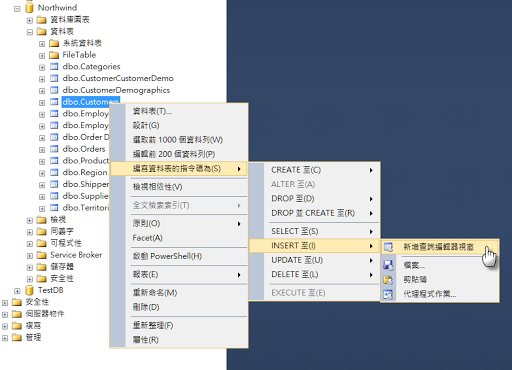## 2016年8月15日 星期一

### 使用 LINQPad 快速產生 Table 的 Insert Script

http://kevintsengtw.blogspot.tw/2015/10/dapper-linqpad-sql-command.html

### 在 SSMS 裡產生 Insert Script`USE [Northwind]`
`GO`
` `
`INSERT INTO [dbo].[Customers]`
`           ([CustomerID]`
`           ,[CompanyName]`
`           ,[ContactName]`
`           ,[ContactTitle]`
`           ,[Address]`
`           ,[City]`
`           ,[Region]`
`           ,[PostalCode]`
`           ,[Country]`
`           ,[Phone]`
`           ,[Fax])`
`     VALUES`
`           (<CustomerID, nchar(5),>`
`           ,<CompanyName, nvarchar(40),>`
`           ,<ContactName, nvarchar(30),>`
`           ,<ContactTitle, nvarchar(30),>`
`           ,<Address, nvarchar(60),>`
`           ,<City, nvarchar(15),>`
`           ,<Region, nvarchar(15),>`
`           ,<PostalCode, nvarchar(10),>`
`           ,<Country, nvarchar(15),>`
`           ,<Phone, nvarchar(24),>`
`           ,<Fax, nvarchar(24),>)`
`GO`
` `
` `

### 使用 LINQPad 產生對映 Table 的 Insert Script

`void Main()`
`{`
`    // 這邊修改為你要執行的 SQL Command`
`    var sqlCommand = @"SELECT top 1 * FROM dbo.Customers WITH (NOLOCK);";`
` `
`    this.Connection.GenerateInsertCommand(sqlCommand.ToString(), "Customer").Dump();`
` `
`}`
` `
`public static class LINQPadExtensions`
`{`
`    public static string GenerateInsertCommand(this IDbConnection connection, string sql, string tableName = "TableName")`
`    {`
`        if (connection.State != ConnectionState.Open)`
`        {`
`            connection.Open();`
`        }`
` `
`        var cmd = connection.CreateCommand();`
`        cmd.CommandText = sql;`
`        var reader = cmd.ExecuteReader();`
` `
`        var builder = new StringBuilder();`
`        do`
`        {`
`            if (reader.FieldCount <= 1)`
`            {`
`                continue;`
`            }`
` `
`            builder.AppendFormat("INSERT INTO [dbo].[{0}]{1}", tableName, Environment.NewLine);`
`            builder.AppendLine("(");`
` `
`            var schema = reader.GetSchemaTable();`
`            var columnNames = new List<string>();`
` `
`            foreach (DataRow row in schema.Rows)`
`            {`
`                var columnName = (string)row["ColumnName"];`
`                columnNames.Add(columnName);`
`            }`
` `
`            foreach (var columnName in columnNames)`
`            {`
`                builder.AppendFormat("    [{0}]{1}{2}",`
`                    columnName,`
`                    columnNames.IndexOf(columnName).Equals(columnNames.Count - 1) ? "" : ",",`
`                    Environment.NewLine);`
`            }`
` `
`            builder.AppendLine(")");`
`            builder.AppendLine("VALUES");`
`            builder.AppendLine("(");`
` `
`            foreach (var columnName in columnNames)`
`            {`
`                builder.AppendFormat("    @{0}{1}{2}",`
`                    columnName,`
`                    columnNames.IndexOf(columnName).Equals(columnNames.Count - 1) ? "" : ",",`
`                    Environment.NewLine);`
`            }`
` `
`            builder.AppendLine(");");`
`            builder.AppendLine();`
`        }`
`        while (reader.NextResult());`
` `
`        return builder.ToString();`
`    }`
`}`

`INSERT INTO [dbo].[Customer]`
`(`
`  [CustomerID],`
`  [CompanyName],`
`  [ContactName],`
`  [ContactTitle],`
`  [Address],`
`  [City],`
`  [Region],`
`  [PostalCode],`
`  [Country],`
`  [Phone],`
`  [Fax]`
`)`
`VALUES`
`(`
`  @CustomerID,`
`  @CompanyName,`
`  @ContactName,`
`  @ContactTitle,`
`  @Address,`
`  @City,`
`  @Region,`
`  @PostalCode,`
`  @Country,`
`  @Phone,`
`  @Fax`
`);`
` `
` `
` `

### 相關連結

mrkt 的程式學習筆記: Dapper - 使用 LINQPad 快速產生相對映 SQL Command 查詢結果的類別

mrkt 的程式學習筆記: Dapper 練習題 - 新增多筆或大量資料